# Using a graphical interface

In the previous tutorial we measured the IV characteristic of a sample to show how we can set up a simple experiment in PyMeasure. The real power of PyMeasure comes when we also use the graphical tools that are included to turn our simple example into a full-fledged user interface.

## Using the Plotter

While it lacks the nice features of the ManagedWindow, the Plotter object is the simplest way of getting live-plotting. The Plotter takes a Results object and plots the data at a regular interval, grabbing the latest data each time from the file.

Warning

The example in this section is known to raise issues when executed: a QApplication was not created in the main thread / nextEventMatchingMask should only be called from the Main Thread warning is raised. While the example works without issues on some operating systems and python configurations, users are advised not to rely on the plotter while this issue is unresolved. Users can hence skip this example and continue with the Using the ManagedWindow section.

Let’s extend our SimpleProcedure with a RandomProcedure, which generates random numbers during our loop. This example does not include instruments to provide a simpler example.

```import logging
log = logging.getLogger(__name__)

import random
from time import sleep
from pymeasure.log import console_log
from pymeasure.display import Plotter
from pymeasure.experiment import Procedure, Results, Worker
from pymeasure.experiment import IntegerParameter, FloatParameter, Parameter

class RandomProcedure(Procedure):

iterations = IntegerParameter('Loop Iterations')
delay = FloatParameter('Delay Time', units='s', default=0.2)
seed = Parameter('Random Seed', default='12345')

DATA_COLUMNS = ['Iteration', 'Random Number']

def startup(self):
log.info("Setting the seed of the random number generator")
random.seed(self.seed)

def execute(self):
log.info("Starting the loop of %d iterations" % self.iterations)
for i in range(self.iterations):
data = {
'Iteration': i,
'Random Number': random.random()
}
self.emit('results', data)
log.debug("Emitting results: %s" % data)
self.emit('progress', 100 * i / self.iterations)
sleep(self.delay)
if self.should_stop():
log.warning("Caught the stop flag in the procedure")
break

if __name__ == "__main__":
console_log(log)

log.info("Constructing a RandomProcedure")
procedure = RandomProcedure()
procedure.iterations = 100

data_filename = 'random.csv'
log.info("Constructing the Results with a data file: %s" % data_filename)
results = Results(procedure, data_filename)

log.info("Constructing the Plotter")
plotter = Plotter(results)
plotter.start()
log.info("Started the Plotter")

log.info("Constructing the Worker")
worker = Worker(results)
worker.start()
log.info("Started the Worker")

log.info("Joining with the worker in at most 1 hr")
worker.join(timeout=3600) # wait at most 1 hr (3600 sec)
log.info("Finished the measurement")
```

The important addition is the construction of the Plotter from the Results object.

```plotter = Plotter(results)
plotter.start()
```

The Plotter is started in a different process so that it can be run on a separate CPU for higher performance. The Plotter launches a Qt graphical interface using pyqtgraph which allows the Results data to be viewed based on the columns in the data.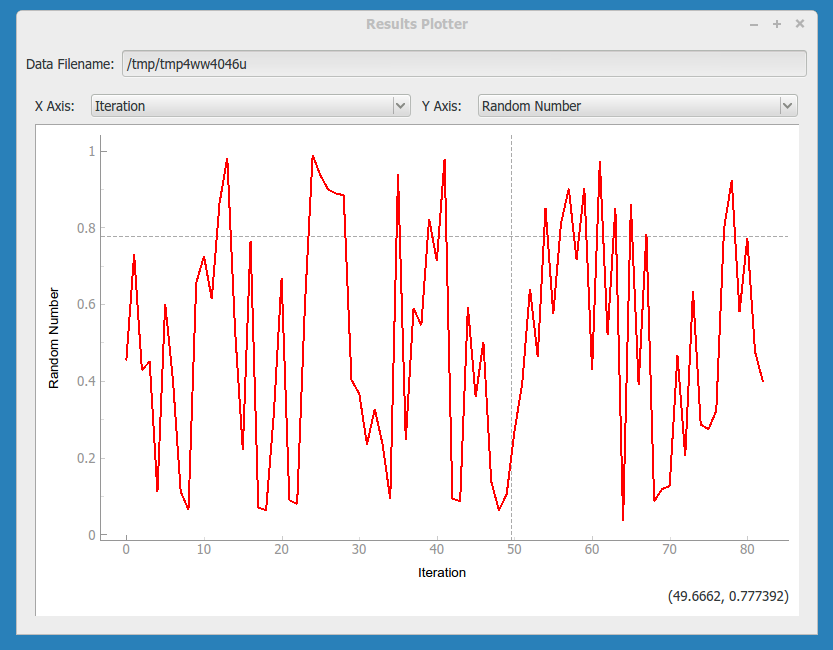## Using the ManagedWindow

The ManagedWindow is the most convenient tool for running measurements with your Procedure. This has the major advantage of accepting the input parameters graphically. From the parameters, a graphical form is automatically generated that allows the inputs to be typed in. With this feature, measurements can be started dynamically, instead of defined in a script.

Another major feature of the ManagedWindow is its support for running measurements in a sequential queue. This allows you to set up a number of measurements with different input parameters, and watch them unfold on the live-plot. This is especially useful for long running measurements. The ManagedWindow achieves this through the Manager object, which coordinates which Procedure the Worker should run and keeps track of its status as the Worker progresses.

Below we adapt our previous example to use a ManagedWindow.

```import logging
log = logging.getLogger(__name__)

import sys
import tempfile
import random
from time import sleep
from pymeasure.log import console_log
from pymeasure.display.Qt import QtWidgets
from pymeasure.display.windows import ManagedWindow
from pymeasure.experiment import Procedure, Results
from pymeasure.experiment import IntegerParameter, FloatParameter, Parameter

class RandomProcedure(Procedure):

iterations = IntegerParameter('Loop Iterations', default=100)
delay = FloatParameter('Delay Time', units='s', default=0.2)
seed = Parameter('Random Seed', default='12345')

DATA_COLUMNS = ['Iteration', 'Random Number']

def startup(self):
log.info("Setting the seed of the random number generator")
random.seed(self.seed)

def execute(self):
log.info("Starting the loop of %d iterations" % self.iterations)
for i in range(self.iterations):
data = {
'Iteration': i,
'Random Number': random.random()
}
self.emit('results', data)
log.debug("Emitting results: %s" % data)
self.emit('progress', 100 * i / self.iterations)
sleep(self.delay)
if self.should_stop():
log.warning("Caught the stop flag in the procedure")
break

class MainWindow(ManagedWindow):

def __init__(self):
super().__init__(
procedure_class=RandomProcedure,
inputs=['iterations', 'delay', 'seed'],
displays=['iterations', 'delay', 'seed'],
x_axis='Iteration',
y_axis='Random Number'
)
self.setWindowTitle('GUI Example')

def queue(self):
filename = tempfile.mktemp()

procedure = self.make_procedure()
results = Results(procedure, filename)
experiment = self.new_experiment(results)

self.manager.queue(experiment)

if __name__ == "__main__":
app = QtWidgets.QApplication(sys.argv)
window = MainWindow()
window.show()
sys.exit(app.exec())
```

This results in the following graphical display.In the code, the MainWindow class is a sub-class of the ManagedWindow class. We override the constructor to provide information about the procedure class and its options. The `inputs` are a list of Parameters class-variable names, which the display will generate graphical fields for. When the list of inputs is long, a boolean key-word argument `inputs_in_scrollarea` is provided that adds a scrollbar to the input area. The `displays` is a list similar to the `inputs` list, which instead defines the parameters to display in the browser window. This browser keeps track of the experiments being run in the sequential queue.

The `queue` method establishes how the Procedure object is constructed. We use the `self.make_procedure` method to create a Procedure based on the graphical input fields. Here we are free to modify the procedure before putting it on the queue. In this context, the Manager uses an Experiment object to keep track of the Procedure, Results, and its associated graphical representations in the browser and live-graph. This is then given to the Manager to queue the experiment.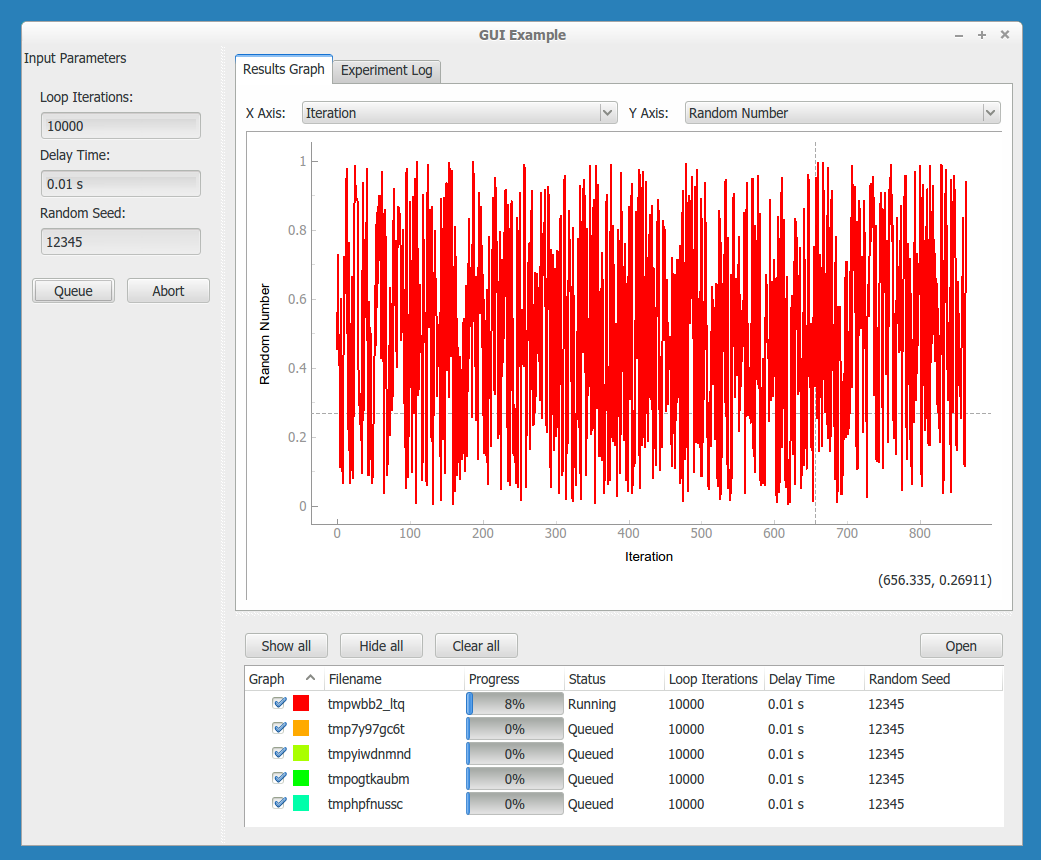By default the Manager starts a measurement when its procedure is queued. The abort button can be pressed to stop an experiment. In the Procedure, the `self.should_stop` call will catch the abort event and halt the measurement. It is important to check this value, or the Procedure will not be responsive to the abort event.If you abort a measurement, the resume button must be pressed to continue the next measurement. This allows you to adjust anything, which is presumably why the abort was needed.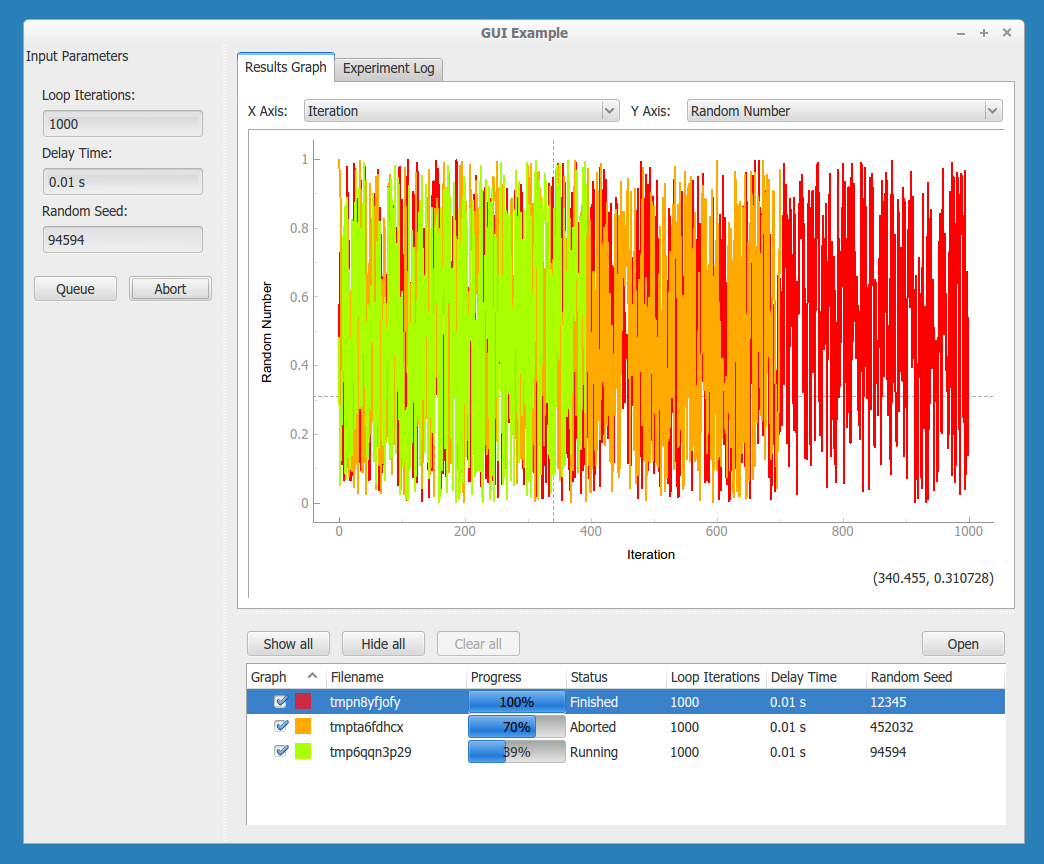Now that you have learned about the ManagedWindow, you have all of the basics to get up and running quickly with a measurement and produce an easy to use graphical interface with PyMeasure.

Note

For performance reasons, the default linewidth of all the graphs has been set to 1. If performance is not an issue, the linewidth can be changed to 2 (or any other value) for better visibility by using the linewidth keyword-argument in the Plotter or the ManagedWindow. Whenever a linewidth of 2 is preferred and a better performance is required, it is possible to enable using OpenGL in the import section of the file:

```import pyqtgraph as pg
pg.setConfigOption("useOpenGL", True)
```

## Customising the plot options

For both the PlotterWindow and ManagedWindow, plotting is provided by the pyqtgraph library. This library allows you to change various plot options, as you might expect: axis ranges (by default auto-ranging), logarithmic and semilogarithmic axes, downsampling, grid display, FFT display, etc. There are two main ways you can do this:

1. You can right click on the plot to manually change any available options. This is also a good way of getting an overview of what options are available in pyqtgraph. Option changes will, of course, not persist across a restart of your program.

2. You can programmatically set these options using pyqtgraph’s PlotItem API, so that the window will open with these display options already set, as further explained below.

For `Plotter`, you can make a sub-class that overrides the `setup_plot()` method. This method will be called when the Plotter constructs the window. As an example

```class LogPlotter(Plotter):
def setup_plot(self, plot):
# use logarithmic x-axis (e.g. for frequency sweeps)
plot.setLogMode(x=True)
```

For `ManagedWindow`, the mechanism to customize plots is much more flexible by using specialization via inheritance. Indeed `ManagedWindowBase` is the base class for `ManagedWindow` and `ManagedImageWindow` which are subclasses ready to use for GUI.

## Using tabular format

In some experiments, data in tabular format may be useful in addition or in alternative to graphical plot. `ManagedWindowBase` allows adding a `TableWidget` to show experiments data, the widget supports also exporting data in some popular format like CSV, HTML, etc. Below an example on how to customize `ManagedWindowBase` to use tabular format, it derived from example above and changed lines are marked.

``` import logging
log = logging.getLogger(__name__)

import sys
import tempfile
import random
from time import sleep
from pymeasure.log import console_log
from pymeasure.display.Qt import QtWidgets
from pymeasure.display.windows import ManagedWindowBase
from pymeasure.display.widgets import TableWidget, LogWidget
from pymeasure.experiment import Procedure, Results
from pymeasure.experiment import IntegerParameter, FloatParameter, Parameter

class RandomProcedure(Procedure):

iterations = IntegerParameter('Loop Iterations', default=10)
delay = FloatParameter('Delay Time', units='s', default=0.2)
seed = Parameter('Random Seed', default='12345')

DATA_COLUMNS = ['Iteration', 'Random Number']

def startup(self):
log.info("Setting the seed of the random number generator")
random.seed(self.seed)

def execute(self):
log.info("Starting the loop of %d iterations" % self.iterations)
for i in range(self.iterations):
data = {
'Iteration': i,
'Random Number': random.random()
}
self.emit('results', data)
log.debug("Emitting results: %s" % data)
self.emit('progress', 100 * i / self.iterations)
sleep(self.delay)
if self.should_stop():
log.warning("Caught the stop flag in the procedure")
break

class MainWindow(ManagedWindowBase):

def __init__(self):
widget_list = (TableWidget("Experiment Table",
RandomProcedure.DATA_COLUMNS,
by_column=True,
),
LogWidget("Experiment Log"),
)
super().__init__(
procedure_class=RandomProcedure,
inputs=['iterations', 'delay', 'seed'],
displays=['iterations', 'delay', 'seed'],
widget_list=widget_list,
)
log.setLevel(self.log_level)
log.info("ManagedWindow connected to logging")
self.setWindowTitle('GUI Example')

def queue(self):
filename = tempfile.mktemp()

procedure = self.make_procedure()
results = Results(procedure, filename)
experiment = self.new_experiment(results)

self.manager.queue(experiment)

if __name__ == "__main__":
app = QtWidgets.QApplication(sys.argv)
window = MainWindow()
window.show()
sys.exit(app.exec())
```

This results in the following graphical display.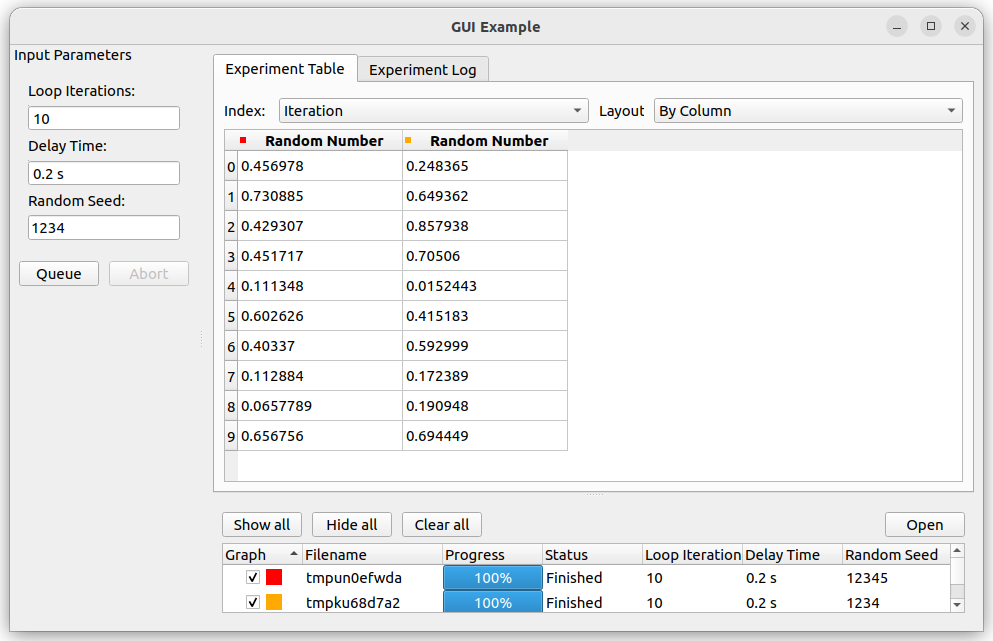## Defining your own ManagedWindow’s widgets

The parameter `widget_list` in `ManagedWindowBase` constructor allow to introduce user’s defined widget in the GUI results display area. The user’s widget should inherit from `TabWidget` and could reimplement any of the methods that needs customization. In order to get familiar with the mechanism, users can check the following widgets already provided:

## Using the sequencer

As an extension to the way of graphically inputting parameters and executing multiple measurements using the `ManagedWindow`, `SequencerWidget` is provided which allows users to queue a series of measurements with varying one, or more, of the parameters. This sequencer thereby provides a convenient way to scan through the parameter space of the measurement procedure.

To activate the sequencer, two additional keyword arguments are added to `ManagedWindow`, namely `sequencer` and `sequencer_inputs`. `sequencer` accepts a boolean stating whether or not the sequencer has to be included into the window and `sequencer_inputs` accepts either `None` or a list of the parameter names are to be scanned over. If no list of parameters is given, the parameters displayed in the manager queue are used.

In order to be able to use the sequencer, the `ManagedWindow` class is required to have a `queue` method which takes a keyword (or better keyword-only for safety reasons) argument `procedure`, where a procedure instance can be passed. The sequencer will use this method to queue the parameter scan.

In order to implement the sequencer into the previous example, only the `ManagedWindow` has to be modified slightly (where modified lines are marked):

``` class MainWindow(ManagedWindow):

def __init__(self):
super().__init__(
procedure_class=TestProcedure,
inputs=['iterations', 'delay', 'seed'],
displays=['iterations', 'delay', 'seed'],
x_axis='Iteration',
y_axis='Random Number',
sequencer_inputs=['iterations', 'delay', 'seed'],    # Added line
)
self.setWindowTitle('GUI Example')

def queue(self, procedure=None):                             # Modified line
filename = tempfile.mktemp()

if procedure is None:                                    # Added line
procedure = self.make_procedure()                    # Indented

results = Results(procedure, filename)
experiment = self.new_experiment(results)

self.manager.queue(experiment)
```

This adds the sequencer underneath the input panel.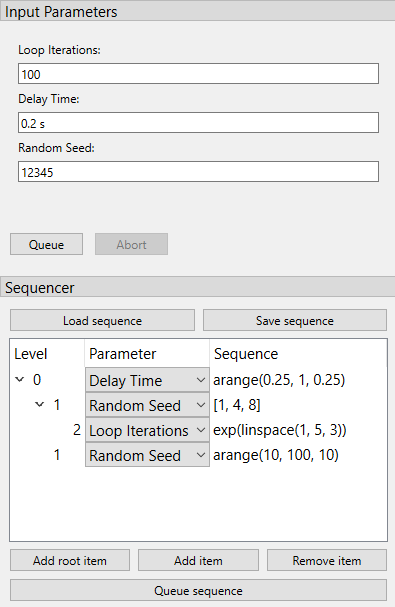The widget contains a tree-view where you can build the sequence. It has three columns: `level` (indicated how deep an item is nested), `parameter` (a drop-down menu to select which parameter is being sequenced by that item), and `sequence` (the text-box where you can define the sequence). While the two former columns are rather straightforward, filling in the later requires some explanation.

In order to maintain flexibility, the sequence is defined in a text-box, allowing the user to enter any list-generating single-line piece of code. To assist in this, a number of functions is supported, either from the main python library (namely `range`, `sorted`, and `list`) or the numpy library. The supported numpy functions (prepending `numpy.` or any abbreviation is not required) are: `arange`, `linspace`, `arccos`, `arcsin`, `arctan`, `arctan2`, `ceil`, `cos`, `cosh`, `degrees`, `e`, `exp`, `fabs`, `floor`, `fmod`, `frexp`, `hypot`, `ldexp`, `log`, `log10`, `modf`, `pi`, `power`, `radians`, `sin`, `sinh`, `sqrt`, `tan`, and `tanh`.

As an example, `arange(0, 10, 1)` generates a list increasing with steps of 1, while using `exp(arange(0, 10, 1))` generates an exponentially increasing list. This way complex sequences can be entered easily.

The sequences can be extended and shortened using the buttons `Add root item`, `Add item`, and `Remove item`. The latter two either add an item as a child of the currently selected item or remove the selected item, respectively. To queue the entered sequence the button `Queue` sequence can be used. If an error occurs in evaluating the sequence text-boxes, this is mentioned in the logger, and nothing is queued.

Finally, it is possible to create a sequence file such that the user does not need to write the sequence again each time. The sequence file can be created by saving current sequence built within the GUI using the `Save sequence` button or directly writing a simple text file. Once created, the sequence can be loaded with the `Load sequence` button.

In the sequence file each line adds one item to the sequence tree, starting with a number of dashes (`-`) to indicate the level of the item (starting with 1 dash for top level), followed by the name of the parameter and the sequence string, both as a python string between parentheses.

An example of such a sequence file is given below, resulting in the sequence shown in the figure above.

```- "Delay Time", "arange(0.25, 1, 0.25)"
-- "Random Seed", "[1, 4, 8]"
--- "Loop Iterations", "exp(linspace(1, 5, 3))"
-- "Random Seed", "arange(10, 100, 10)"
```

This file can also be automatically loaded at the start of the program by adding the key-word argument `sequence_file="filename.txt"` to the `super().__init__` call, as was done in the example.

## Using the directory input

It is possible to add a directory input in order to choose where the experiment’s result will be saved. This option is activated by passing a boolean key-word argument `directory_input` during the `ManagedWindow` init. The value of the directory can be retrieved and set using the property `directory`. A default directory can be defined by setting the `directory` property in the MainWindow init.

Only the MainWindow needs to be modified in order to use this option (modified lines are marked).

``` class MainWindow(ManagedWindow):

def __init__(self):
super().__init__(
procedure_class=TestProcedure,
inputs=['iterations', 'delay', 'seed'],
displays=['iterations', 'delay', 'seed'],
x_axis='Iteration',
y_axis='Random Number',
directory_input=True,                                # Added line, enables directory widget
)
self.setWindowTitle('GUI Example')
self.directory = r'C:/Path/to/default/directory'         # Added line, sets default directory for GUI load

def queue(self):
directory = self.directory                               # Added line
filename = unique_filename(directory)                    # Modified line

results = Results(procedure, filename)
experiment = self.new_experiment(results)

self.manager.queue(experiment)
```

This adds the input line above the Queue and Abort buttons.A completer is implemented allowing to quickly select an existing folder, and a button on the right side of the input widget opens a browse dialog.

## Using the estimator widget

In order to provide estimates of the measurement procedure, an `EstimatorWidget` is provided that allows the user to define and calculate estimates. The widget is automatically activated when the `get_estimates` method is added in the `Procedure`.

The quickest and most simple implementation of the `get_estimates` function simply returns the estimated duration of the measurement in seconds (as an `int` or a `float`). As an example, in the example provided in the Using the ManagedWindow section, the `Procedure` is changed to:

```class RandomProcedure(Procedure):

# ...

def get_estimates(self, sequence_length=None, sequence=None):

return self.iterations * self.delay
```

This will add the estimator widget at the dock on the left. The duration and finishing-time of a single measurement is always displayed in this case. Depending on whether the SequencerWidget is also used, the length, duration and finishing-time of the full sequence is also shown.

For maximum flexibility (e.g. for showing multiple and other types of estimates, such as the duration, filesize, finishing-time, etc.) it is also possible that the `get_estimates` returns a list of tuples. Each of these tuple consists of two strings: the first is the name (label) of the estimate, the second is the estimate itself.

As an example, in the example provided in the Using the ManagedWindow section, the `Procedure` is changed to:

```class RandomProcedure(Procedure):

# ...

def get_estimates(self, sequence_length=None, sequence=None):

duration = self.iterations * self.delay

estimates = [
("Duration", "%d s" % int(duration)),
("Number of lines", "%d" % int(self.iterations)),
("Sequence length", str(sequence_length)),
('Measurement finished at', str(datetime.now() + timedelta(seconds=duration))),
]

return estimates
```

This will add the estimator widget at the dock on the left.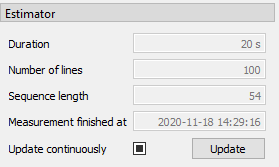Note that after the initialisation of the widget both the label of the estimate as of course the estimate itself can be modified, but the amount of estimates is fixed.

The keyword arguments are not required in the implementation of the function, but are passed if asked for (i.e. `def get_estimates(self)` does also works). Keyword arguments that are accepted are `sequence`, which contains the full sequence of the sequencer (if present), and `sequence_length`, which gives the length of the sequence as integer (if present). If the sequencer is not present or the sequence cannot be parsed, both `sequence` and `sequence_length` will contain `None`.

The estimates are automatically updated every 2 seconds. Changing this update interval is possible using the “Update continuously”-checkbox, which can be toggled between three states: off (i.e. no updating), auto-update every two seconds (default) or auto-update every 100 milliseconds. Manually updating the estimates (useful whenever continuous updating is turned off) is also possible using the “update”-button.

## Flexible hiding of inputs

There can be situations when it may be relevant to turn on or off a number of inputs (e.g. when a part of the measurement script is skipped upon turning of a single `BooleanParameter`). For these cases, it is possible to assign a `Parameter` to a controlling `Parameter`, which will hide or show the `Input` of the `Parameter` depending on the value of the `Parameter`. This is done with the `group_by` key-word argument.

```toggle = BooleanParameter("toggle", default=True)
param = FloatParameter('some parameter', group_by='toggle')
```

When both the `toggle` and `param` are visible in the `InputsWidget` (via `inputs=['iterations', 'delay', 'seed']` as demonstrated above) one can control whether the input-field of `param` is visible by checking and unchecking the checkbox of `toggle`. By default, the group will be visible if the value of the `group_by` `Parameter` is `True` (which is only relevant for a `BooleanParameter`), but it is possible to specify other value as conditions using the `group_condition` keyword argument.

```iterations = IntegerParameter('Loop Iterations', default=100)
param = FloatParameter('some parameter', group_by='iterations', group_condition=99)
```

Here the input of `param` is only visible if `iterations` has a value of 99. This works with any type of `Parameter` as `group_by` parameter.

To allow for even more flexibility, it is also possible to pass a (lambda)function as a condition:

```iterations = IntegerParameter('Loop Iterations', default=100)
param = FloatParameter('some parameter', group_by='iterations', group_condition=lambda v: 50 < v < 100)
```

Now the input of `param` is only shown if the value of `iterations` is between 51 and 99.

Using the `hide_groups` keyword-argument of the `ManagedWindow` you can choose between hiding the groups (`hide_groups = True`) and disabling / graying-out the groups (`hide_groups = False`).

Finally, it is also possible to provide multiple parameters to the `group_by` argument, in which case the input will only be visible if all of the conditions are true. Multiple parameters for grouping can either be passed as a dict of string: condition pairs, or as a list of strings, in which case the `group_condition` can be either a single condition or a list of conditions:

```iterations = IntegerParameter('Loop Iterations', default=100)
toggle = BooleanParameter('A checkbox')
param_A = FloatParameter('some parameter', group_by=['iterations', 'toggle'], group_condition=[lambda v: 50 < v < 100, True])
param_B = FloatParameter('some parameter', group_by={'iterations': lambda v: 50 < v < 100, 'toggle': True})
```

Note that in this example, `param_A` and `param_B` are identically grouped: they’re only visible if `iterations` is between 51 and 99 and if the toggle checkbox is checked (i.e. True).

## Using the ManagedDockWindow

Building off the Using the ManagedWindow section where we used a `ManagedWindow`, we can also use `ManagedDockWindow` to build a graphical interface with multiple graphs that can be docked in the main GUI window or popped out into their own window.

To start with, let’s make the following highlighted edits to the code example from Using the ManagedWindow:

1. On line 10 we now import `ManagedDockWindow`

2. On line 20, and lines 32 and 33, we add two new columns of data to be recorded `'Random Number 2'` and `'Random Number 3'`

3. On line 44 we make `MainWindow` a subclass of `ManagedDockWindow`

4. On line 51 we will pass in a list of strings from `DATA_COLUMNS` to the `x_axis` argument

5. On line 52 we will pass in a list of strings from `DATA_COLUMNS` to the `y_axis` argument

```import logging
log = logging.getLogger(__name__)

import sys
import tempfile
import random
from time import sleep
from pymeasure.display.Qt import QtWidgets
from pymeasure.display.windows.managed_dock_window import ManagedDockWindow
from pymeasure.experiment import Procedure, Results
from pymeasure.experiment import IntegerParameter, FloatParameter, Parameter

class RandomProcedure(Procedure):

iterations = IntegerParameter('Loop Iterations', default=10)
delay = FloatParameter('Delay Time', units='s', default=0.2)
seed = Parameter('Random Seed', default='12345')

DATA_COLUMNS = ['Iteration', 'Random Number 1', 'Random Number 2', 'Random Number 3']

def startup(self):
log.info("Setting the seed of the random number generator")
random.seed(self.seed)

def execute(self):
log.info("Starting the loop of %d iterations" % self.iterations)
for i in range(self.iterations):
data = {
'Iteration': i,
'Random Number 1': random.random(),
'Random Number 2': random.random(),
'Random Number 3': random.random()
}
self.emit('results', data)
log.debug("Emitting results: %s" % data)
self.emit('progress', 100 * i / self.iterations)
sleep(self.delay)
if self.should_stop():
log.warning("Caught the stop flag in the procedure")
break

class MainWindow(ManagedDockWindow):

def __init__(self):
super().__init__(
procedure_class=RandomProcedure,
inputs=['iterations', 'delay', 'seed'],
displays=['iterations', 'delay', 'seed'],
x_axis=['Iteration', 'Random Number 1'],
y_axis=['Random Number 1','Random Number 2', 'Random Number 3']
)
self.setWindowTitle('GUI Example')

def queue(self):
filename = tempfile.mktemp()

procedure = self.make_procedure()
results = Results(procedure, filename)
experiment = self.new_experiment(results)

self.manager.queue(experiment)

if __name__ == "__main__":
app = QtWidgets.QApplication(sys.argv)
window = MainWindow()
window.show()
sys.exit(app.exec())
```

Now we can see our `ManagedDockWindow`: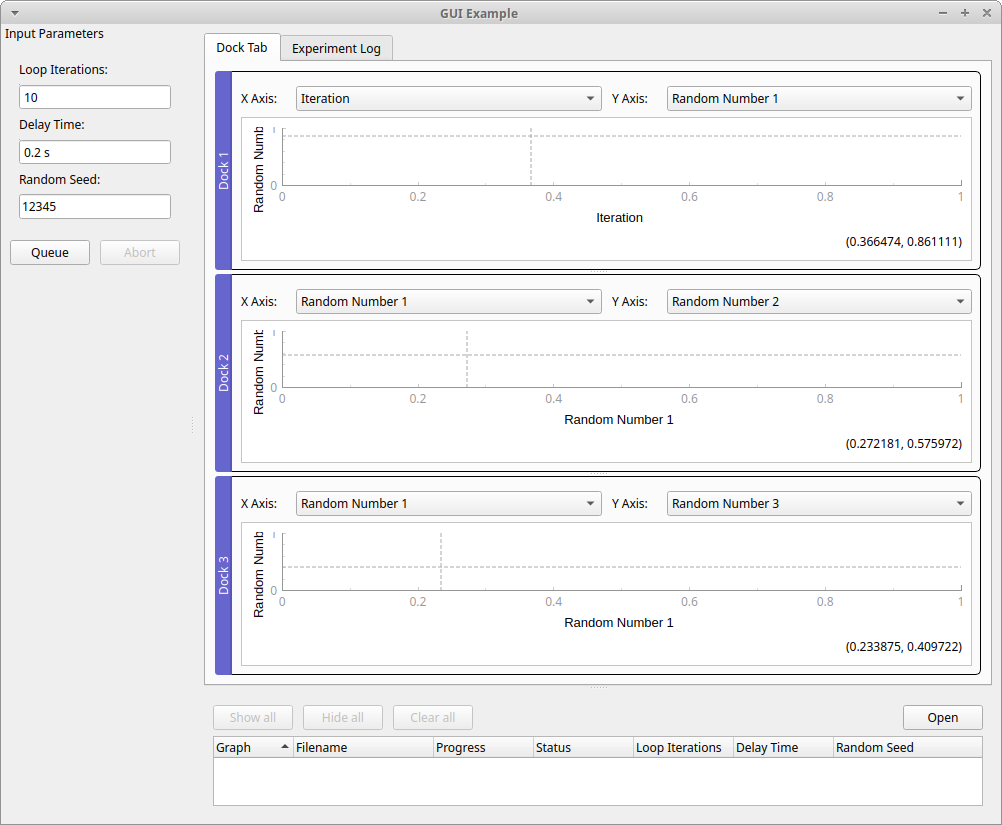As you can see from the above screenshot, our example code created three docks with following “X Axis” and “Y Axis” labels:

1. X Axis: “Iteration” Y Axis: “Random Number 1”

2. X Axis: “Random Number 1” Y Axis: “Random Number 2”

3. X Axis: “Random Number 1” Y Axis: “Random Number 3”

The list of strings for `x_axis` and `y_axis` set the default labels for each dockable plot and the longest list determines how many dockable plots are created. To highlight this point, in our example we define `x_axis` and `y_axis` with the following lists:

```x_axis=['Iteration', 'Random Number 1'],
y_axis=['Random Number 1','Random Number 2', 'Random Number 3']
```

If one list is longer than the last element if the other list is used as the default label for the rest of the dockable plots. In our example that is why we have two X Axis labels with “Random Number 1”. The longest list between `x_axis` and `y_axis` determines the number of plots. In our example `y_axis` has the longest list with a length of three so three plots are created.

You can pop out a dockable plot from the main dock window to its own window by double clicking the blue “Dock #” title bar, which is to the left of each plot by default: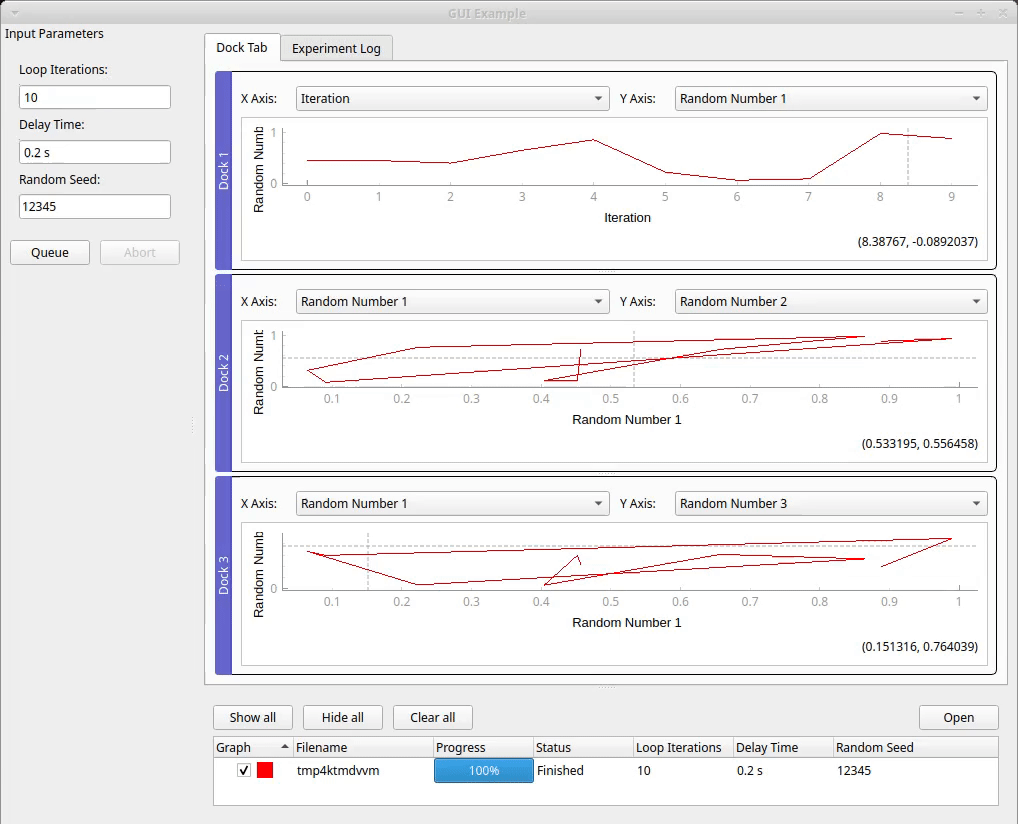You can return the popped out window to the main window by clicking the close icon X in the top right.

After positioning your dock windows, you can save the layout by right-clicking a dock widget and select “Save Dock Layout” from the context menu. This will save the layout of all docks and the settings for each plot to a file. By default the file path is the current working directory of the python file that started `ManagedDockWindow`, and the default file name is ‘procedure class + “_dock_layout.json”’. For our example, that would be “./RandomProcedure_dock_layout.json”

When you run the python file that invokes `ManagedDockWindow` again, it will look for and load the dock layout file if it exists.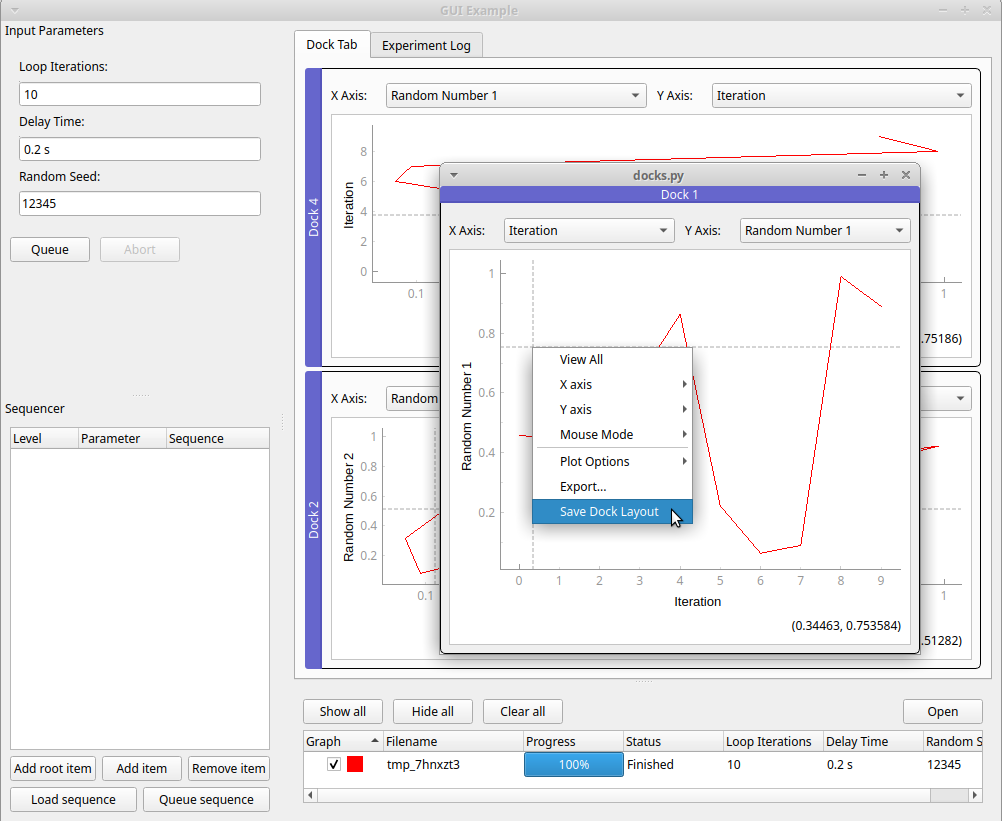You can drag a dockable plot to reposition it in reference to other plots in the main dock window in several ways. You can drag the blue “Dock #” title bar to the left or right side of another plot to reposition a plot to be side by side with another plot: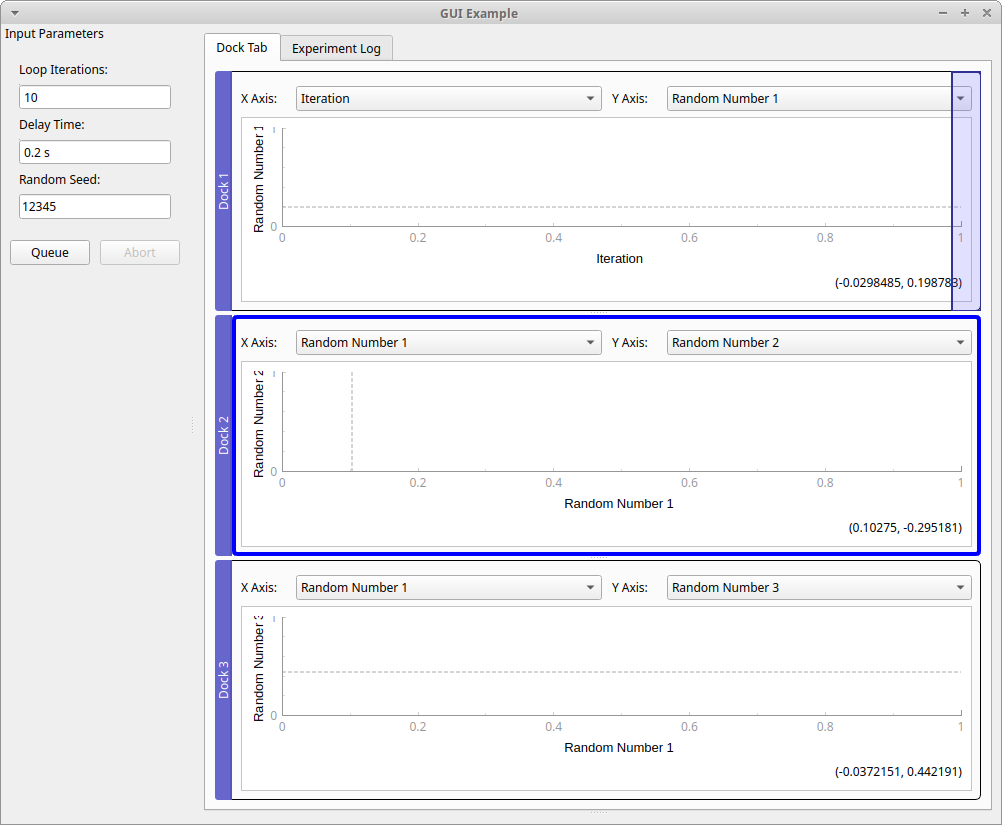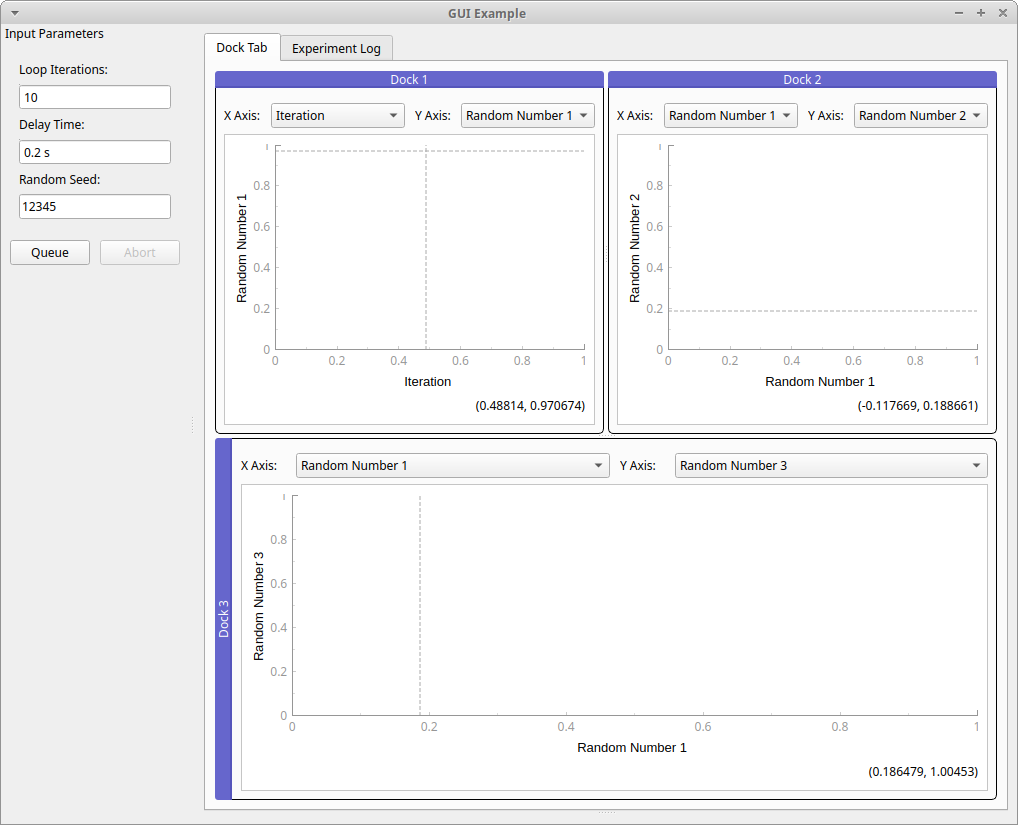You can also drag the blue “Dock #” title bar to the top or bottom side of another plot to reposition a plot to rearrange the vertical order of the plots: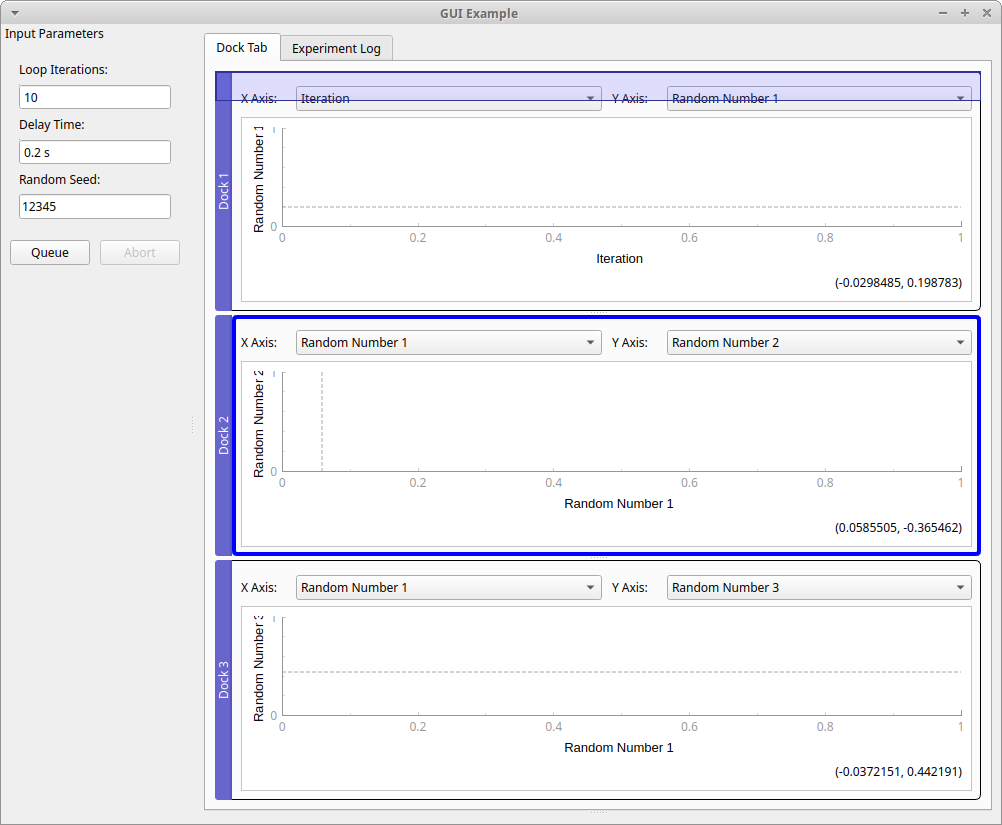You can drag the blue “Dock #” title bar to the middle of another plot to reposition a plot to create a tabbed view of the two plots: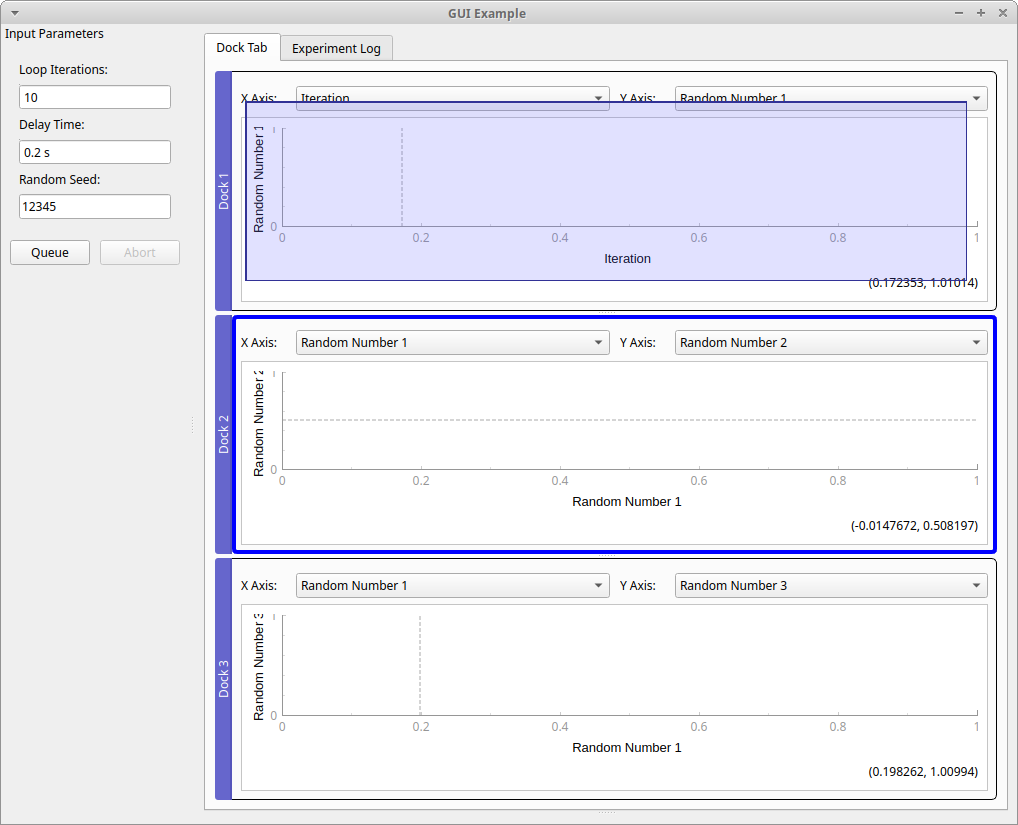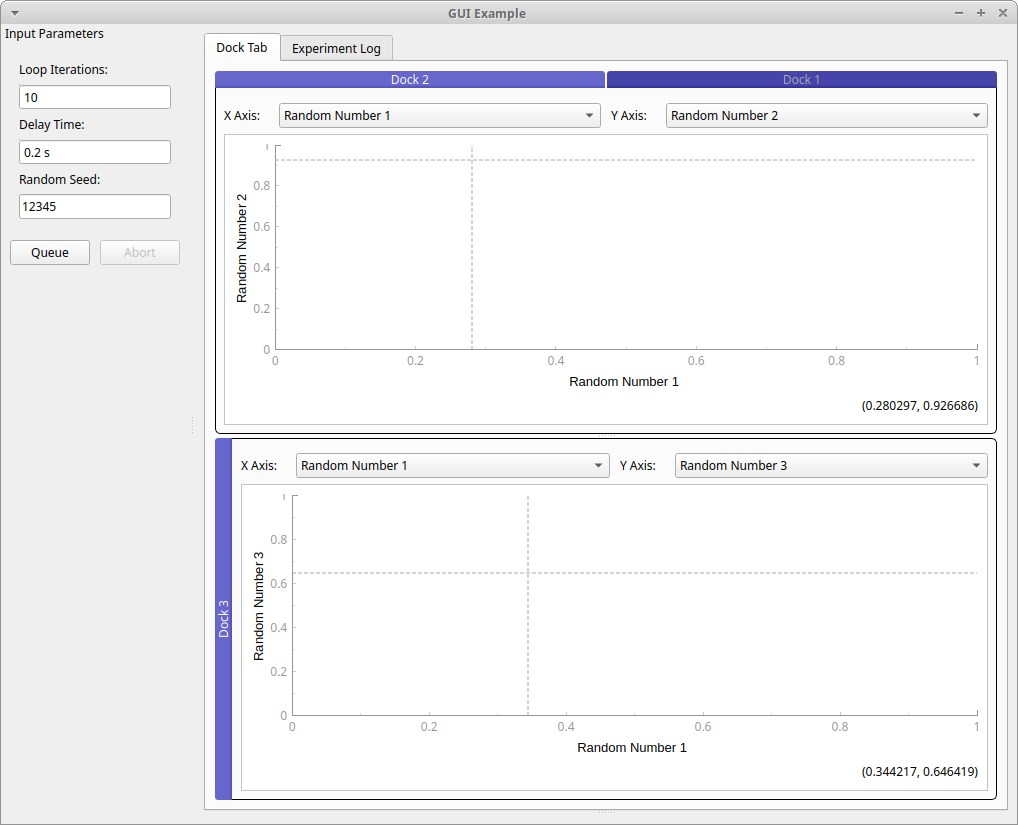## Using the ManagedConsole

The `ManagedConsole` is the most convenient tool for running measurements with your Procedure using a command line interface. The `ManagedConsole` allows to run an experiment with the same set of parameters available in the `ManagedWindow`, but they are defined using a set of command line switches.

It is also possible to define a test that uses both `ManagedConsole` or `ManagedWindow` according to user selection in the command line.

Enabling console mode is easy and straightforward and the following example demonstrates how to do it.

The following example is a variant of the code example from Using the ManagedWindow where some parts have been highlighted:

1. On line 8 we now import `ManagedConsole`

2. On line 73, we add the support for console mode

```import sys
import random
import tempfile
from time import sleep

from pymeasure.experiment import Procedure, IntegerParameter, Parameter, FloatParameter
from pymeasure.experiment import Results
from pymeasure.display.console import ManagedConsole
from pymeasure.display.Qt import QtWidgets
from pymeasure.display.windows import ManagedWindow
import logging

log = logging.getLogger('')

class TestProcedure(Procedure):
iterations = IntegerParameter('Loop Iterations', default=100)
delay = FloatParameter('Delay Time', units='s', default=0.2)
seed = Parameter('Random Seed', default='12345')

DATA_COLUMNS = ['Iteration', 'Random Number']

def startup(self):
log.info("Setting up random number generator")
random.seed(self.seed)

def execute(self):
log.info("Starting to generate numbers")
for i in range(self.iterations):
data = {
'Iteration': i,
'Random Number': random.random()
}
log.debug("Produced numbers: %s" % data)
self.emit('results', data)
self.emit('progress', 100 * (i + 1) / self.iterations)
sleep(self.delay)
if self.should_stop():
log.warning("Catch stop command in procedure")
break

def shutdown(self):
log.info("Finished")

class MainWindow(ManagedWindow):

def __init__(self):
super(MainWindow, self).__init__(
procedure_class=TestProcedure,
inputs=['iterations', 'delay', 'seed'],
displays=['iterations', 'delay', 'seed'],
x_axis='Iteration',
y_axis='Random Number'
)
self.setWindowTitle('GUI Example')

def queue(self):
filename = tempfile.mktemp()

procedure = self.make_procedure()
results = Results(procedure, filename)
experiment = self.new_experiment(results)

self.manager.queue(experiment)

if __name__ == "__main__":
if len(sys.argv) > 1:
# If any parameter is passed, the console mode is run
# This criteria can be changed at user discretion
app = ManagedConsole(procedure_class=TestProcedure)
else:
app = QtWidgets.QApplication(sys.argv)
window = MainWindow()
window.show()

sys.exit(app.exec())
```

If we run the script above without any parameter, you will have the graphical user interface example. If you run as follow, you will use the command line mode:

```python console.py --iterations 10 --result-file console_test
```

Console output is as follow (to show the progress bar, you need to install the optional module progressbar2):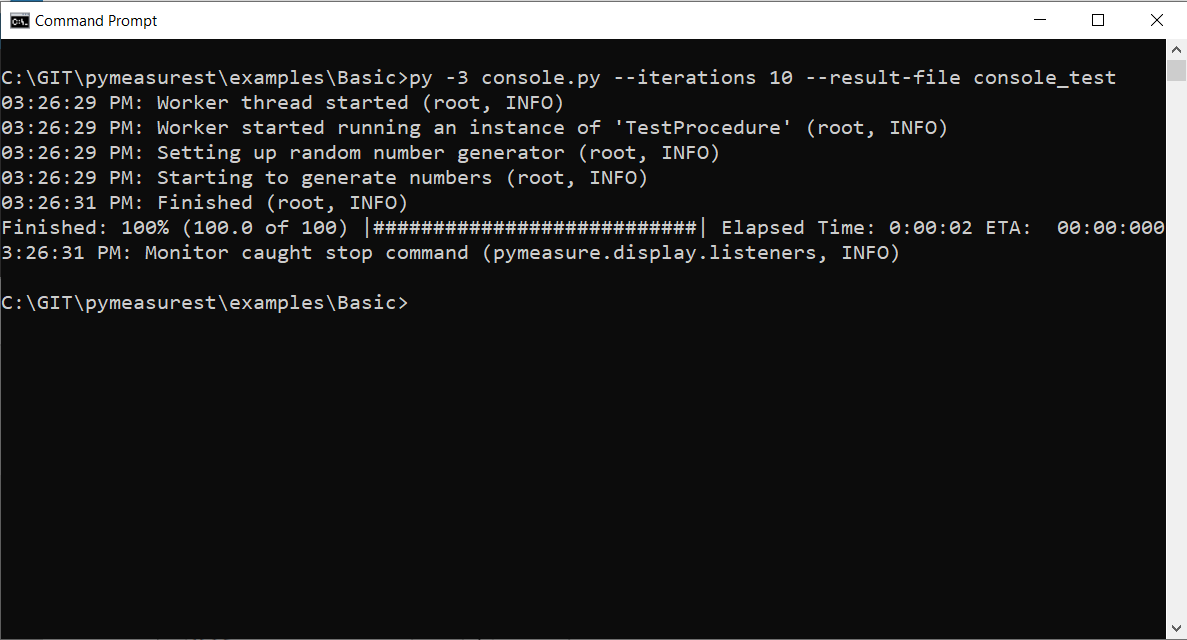### Other useful commands

To show all the command line switches:

```python console.py --help
```

To run an experiment with parameters retrieved from an existing result file.

```python console.py --use-result-file console_test2023-08-09_1.csv
```